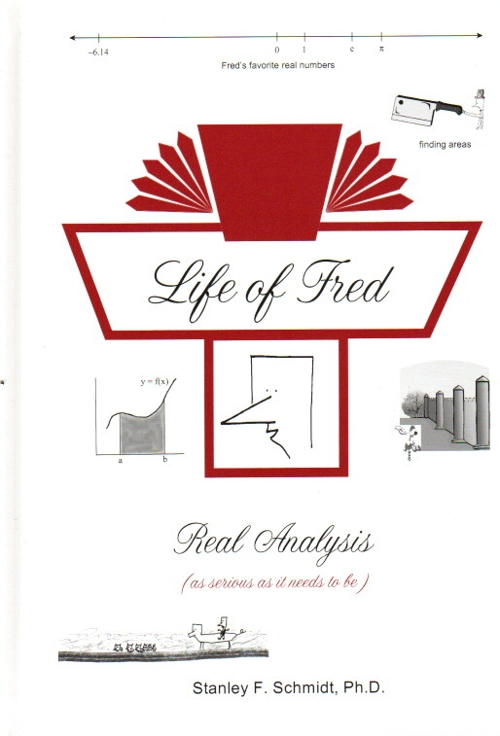Life of Fred Real Analysis \$49 : The Real Numbers, Sequences, Series, Tests for Series Convergence, Limits and Continuity, Derivatives, the Riemann Integral, Sequences of Functions, Series of Functions, and Looking Ahead to Topics beyond a First Course in Real Analysis. Subtopics include: The axiomatic approach to the real numbers, eleven properties of the real numbers, mathematics after calculus, definition of a function, if a and b are irrational, must ab also be irrational?, two definitions of dense subsets, the natural numbers are well-ordered, the positive real numbers are Archimedean—two definitions, math induction proofs, one-to-one (injective) functions, cardinality of a set, four definitions of onto, finding a one-to-one onto function from (0, 1) to [0, 1], countable and uncountable sets, Root Test, Ratio Test, Integral Test, absolute and conditional convergence, weak and strong induction proofs, secant lines, limit proofs using ε and δ, eight theorems about limits and their proofs, lim g(f(x)) does not always equal g(lim f(x)), continuous functions, four theorems about pairs of continuous functions, the squeeze theorem, a very short proof that lim sin x = 0 as x approaches zero, two definitions of derivative, the delta process, the five standard derivative rules and their proofs, how much detail to put in a proof, Schwarzschild radii, converses, contrapositives, and inverses, Intermediate Value Theorem, Rolle’s theorem, Mean Value Theorem, L’Hospital’s rule, proving lim (sin θ)/θ = 1 in two steps, detailed definition of the Riemann integral, uniform continuity, Fundamental Theorem of Calculus, Cauchy sequence of functions, Cauchy series of functions, uniform convergence of a series of functions, Weierstrass M-test, power series, two formulas for the radius of convergence, taking derivatives and antiderivatives of a power series, Weierstrass Approximation theorem, finding an approximation for ln 5 on a desert island, and the Cantor set. ISBN: 978-1-937032-52-4, hardback, 304 pages. \$49